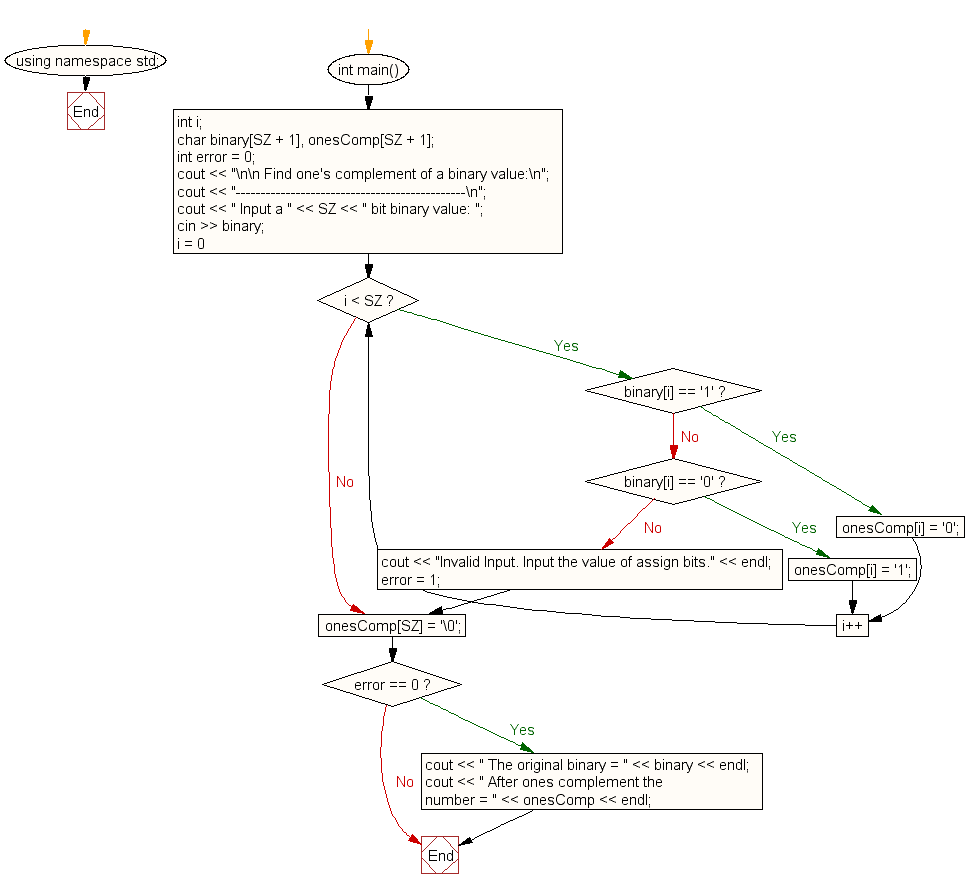﻿ C++ Exercises: Find one's complement of a binary number - w3resource

# C++ Exercises: Find one's complement of a binary number

## C++ For Loop: Exercise-64 with Solution

Write a program in C++ to find the one's complement of a binary number.

Sample Solution:-

C++ Code :

``````#include <iostream>
#define SZ 8
using namespace std;
int main()
{
int i;
char binary[SZ + 1], onesComp[SZ + 1];
int error = 0;
cout << "\n\n Find one's complement of a binary value:\n";
cout << "----------------------------------------------\n";
cout << " Input a " << SZ << " bit binary value: ";
cin >> binary;
for (i = 0; i < SZ; i++)
{
if (binary[i] == '1')
{
onesComp[i] = '0';
}
else if (binary[i] == '0')
{
onesComp[i] = '1';
}
else
{
cout << "Invalid Input. Input the value of assign bits." << endl;
error = 1;
break;
}
}
onesComp[SZ] = '\0';
if (error == 0)
{
cout << " The original binary = " << binary << endl;
cout << " After ones complement the number = " << onesComp << endl;
}
}
``````

Sample Output:

``` Find one's complement of a binary value:
----------------------------------------------
Input a 8 bit binary value: 10100101
The original binary = 10100101
After ones complement the number = 01011010
```

Flowchart:C++ Code Editor: